# 一起来写个简单的解释器（12）

“不怕慢， 只怕停。“ ——中国谚语。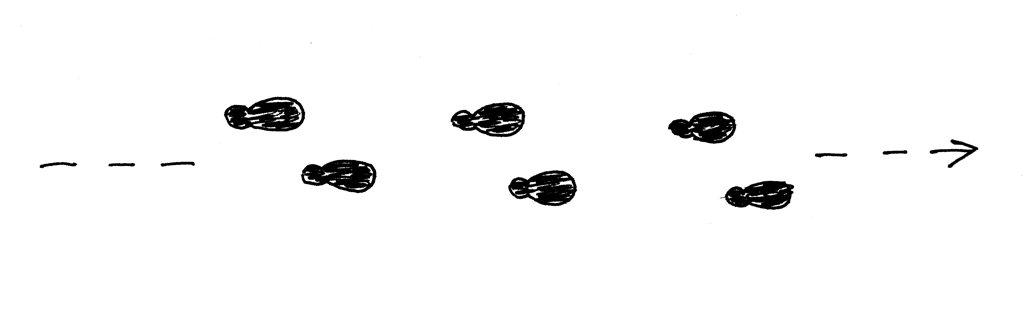• Pascal过程没有返回语句。 当到达相应区块的结束位置时，它们退出。
• Pascal过程可以嵌套在一起。
• 为了简单起见，本文中的过程声明不会有任何形式参数。 但别担心，我们将在后面的章节中介绍。

``````PROGRAM Part12;
VAR
a : INTEGER;

PROCEDURE P1;
VAR
a : REAL;
k : INTEGER;

PROCEDURE P2;
VAR
a, z : INTEGER;
BEGIN {P2}
z := 777;
END;  {P2}

BEGIN {P1}

END;  {P1}

BEGIN {Part12}
a := 10;
END.  {Part12}
``````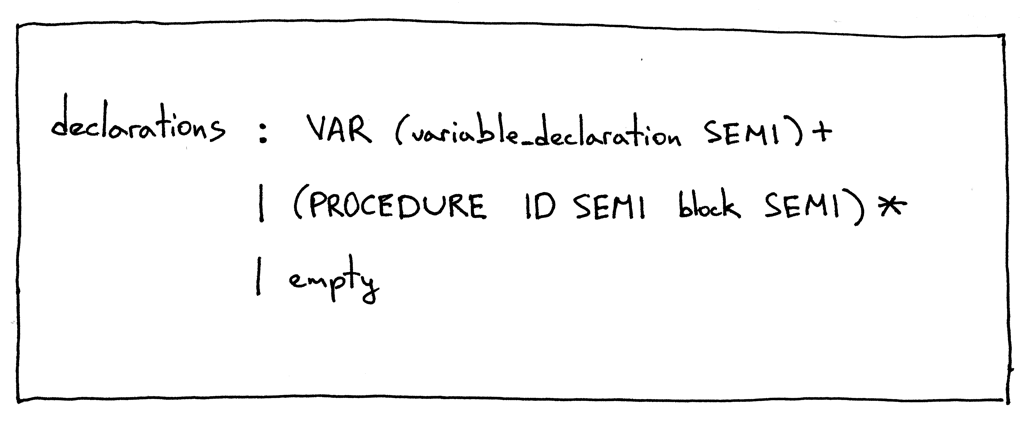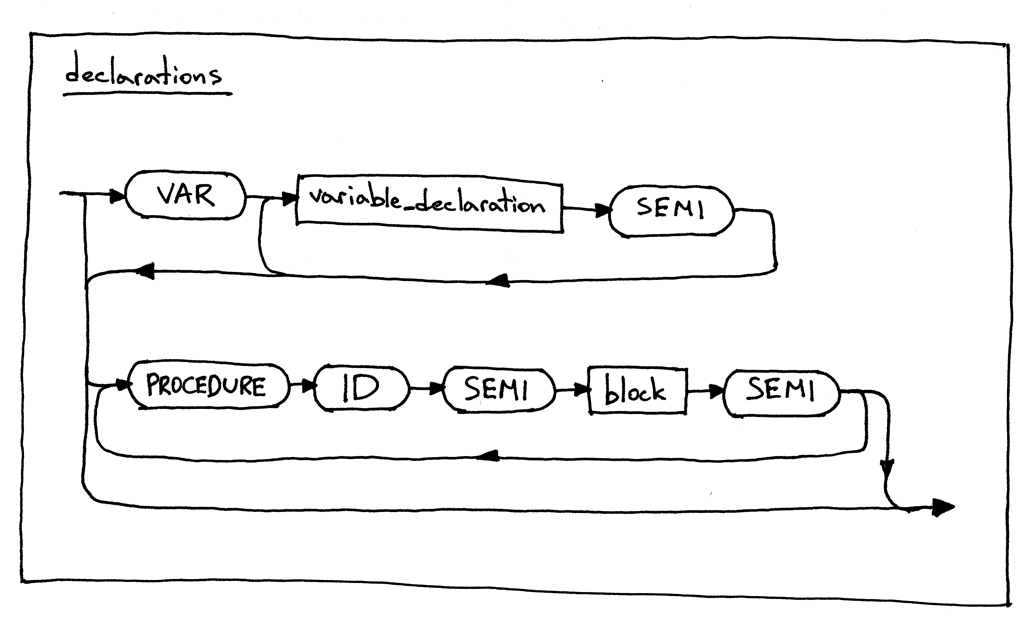``````PROGRAM Test;
VAR
a : INTEGER;

PROCEDURE P1;
BEGIN {P1}

END;  {P1}

PROCEDURE P1A;
BEGIN {P1A}

END;  {P1A}

BEGIN {Test}
a := 10;
END.  {Test}
``````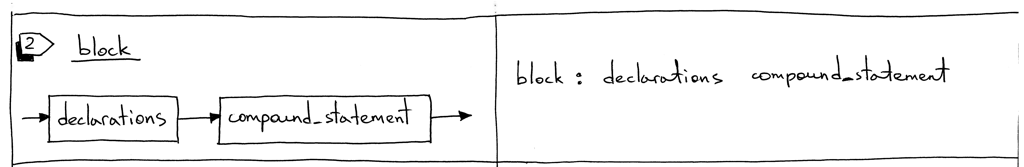``````PROCEDURE = 'PROCEDURE'
``````

``````RESERVED_KEYWORDS = {
'PROGRAM': Token('PROGRAM', 'PROGRAM'),
'VAR': Token('VAR', 'VAR'),
'DIV': Token('INTEGER_DIV', 'DIV'),
'INTEGER': Token('INTEGER', 'INTEGER'),
'REAL': Token('REAL', 'REAL'),
'BEGIN': Token('BEGIN', 'BEGIN'),
'END': Token('END', 'END'),
'PROCEDURE': Token('PROCEDURE', 'PROCEDURE'),
}
``````

1. 新的ProcedureDecl AST节点
2. 更新解析器的声明方法以支持过程声明

1. ProcedureDecl AST节点表示一个过程声明。 类构造函数将过程名称和过程引用代码块的AST节点作为参数。

``````class ProcedureDecl(AST):
def __init__(self, proc_name, block_node):
self.proc_name = proc_name
self.block_node = block_node
``````
2. 这里是Parser类的更新的 declarations 方法

``````def declarations(self):
"""declarations : VAR (variable_declaration SEMI)+
| (PROCEDURE ID SEMI block SEMI)*
| empty
"""
declarations = []

if self.current_token.type == VAR:
self.eat(VAR)
while self.current_token.type == ID:
var_decl = self.variable_declaration()
declarations.extend(var_decl)
self.eat(SEMI)

while self.current_token.type == PROCEDURE:
self.eat(PROCEDURE)
proc_name = self.current_token.value
self.eat(ID)
self.eat(SEMI)
block_node = self.block()
proc_decl = ProcedureDecl(proc_name, block_node)
declarations.append(proc_decl)
self.eat(SEMI)

return declarations
``````

希望上面的代码是不言自明的。 它遵循本文前面的过程声明的语法/语法图。

``````def visit_ProcedureDecl(self, node):
pass
``````

``````PROGRAM Part12;
VAR
a : INTEGER;

PROCEDURE P1;
VAR
a : REAL;
k : INTEGER;

PROCEDURE P2;
VAR
a, z : INTEGER;
BEGIN {P2}
z := 777;
END;  {P2}

BEGIN {P1}

END;  {P1}

BEGIN {Part12}
a := 10;
END.  {Part12}
``````

``````\$ python genastdot.py part12.pas > ast.dot && dot -Tpng -o ast.png ast.dot
``````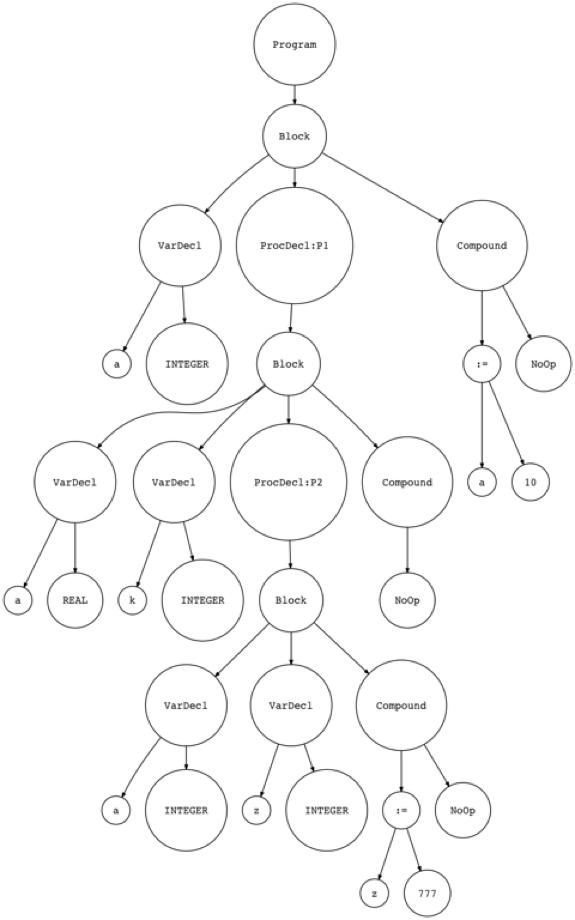``````\$ python spi.py part12.pas
Define: INTEGER
Define: REAL
Lookup: INTEGER
Define: <a:INTEGER>
Lookup: a

Symbol Table contents:
Symbols: [INTEGER, REAL, <a:INTEGER>]

Run-time GLOBAL_MEMORY contents:
a = 10
``````